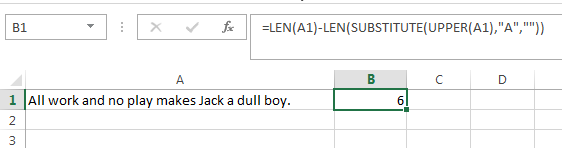# Count Number of Times a Character Appears in Cell

Yesterday I got an email from one of my readers saying that he wanted to count the number of instances of a character inside a cell.

To give you a better understanding of the question let me explain the question in detail.

For instance: The A1 cell of your excel sheet contains a text string like “All work and no play makes Jack a dull boy” now the reader wants to know how many times the character ‘a’ is used in this cell.After thinking for a few minutes I came up with a formula. And today I will explain to my readers how they can deal with such scenarios.

## Finding the Count of a Specific Character in a Cell

To deal with this scenario we can use the formula:

`=LEN(A1)-LEN(SUBSTITUTE(A1,"a",""))`

### The Logic Behind this formula

The main idea around which this formula revolves is the character counting. If you remove all the instances of character ‘a’ from the given string then you are left with a string whose length is lesser than the original string. Now if you subtract the above string length (without a’s) with the original string length (with a’s) then their difference would be equal to the number of a’s in the string.

### What does this formula do?

This formula is made up of three segments and therefore I will explain each one of them separately:

• Part 1: This part constitutes the formula `SUBSTITUTE(A1,"a","")`. If you are already aware of the SUBSTITUTE function then you can easily judge what this function is doing here. For those who are unaware of the SUBSTITUTE function, this function has the only job of replacing all the instances of character ‘a’ with “”.
• Part 2: The second part constitutes the formula `LEN(SUBSTITUTE(A1,"a",""))`. LEN function is an excel formula that counts the number of characters in a text string. So, here this function counts the number of characters in the Part 1 string (i.e. original string without a’s)
• Part 3: The third and the last part constitutes the formula `LEN(A1)`. The job of this formula is to count the number of characters in the original text string.

Finally, the result of the whole formula is the result of Part3 – Part2.### What’s the result?

When the above formula is applied on the source string then it results into 5

But actually, there are 6 a’s in the above string.

### So, what’s the catch?

The catch is that this formula is differentiating between ‘a’ and ‘A’. So, it does not counts the upper case ‘A’ while resulting in the number of ‘a’ characters. And hence it is not counting the first ‘A’.

### How to avoid this catch

This problem can be avoided by simply modifying the above formula as:

`=LEN(A1)-LEN(SUBSTITUTE(LOWER(A1),"a",""))`

You will notice that inside the SUBSTITUTE function I have used another formula LOWER. LOWER function converts all the characters of the source string to lower case and hence now this formula can count both the cases.Another very obvious alternative, to the above formula, can be:

`=LEN(A1)-LEN(SUBSTITUTE(UPPER(A1),"a",""))`

Here, we are converting all the source sting characters to upper case and then we are counting them.About Ankit Kaul

Ankit is the founder of Excel Trick. He is tech Geek who loves to sit in front of his square headed girlfriend (his PC) all day long. :D. Ankit has a strong passion for learning Microsoft Excel. His only aim is to turn you guys into 'Excel Geeks'.